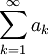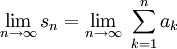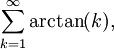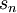# Introduction to the Divergence Test

Previous: The Divergence Test

Next: A Useful Theorem

In previous lessons, we defined the concept of the convergence of an infinite series: the infinite seriesconverges if and only if the limitexists. As was mentioned, it can be very difficult to apply this limit directly, leading us to the question: is there an easier way to determine if an infinite series converges or diverges?

## Examples

Consider for exampleor perhapsThere might be a way of finding an explicit formula forfor these series, and with these formula we may be able to use the definition of convergence directly to establish whether or not these series converge.

But, there is an easier way that quickly tells us that they do not. We can use the divergence test.

Previous: The Divergence Test

Next: A Useful Theorem